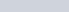• 2
•
•
•
• 1
•
•
•
3
Shares

# SPEED, DISTANCE, AND TIME PROBLEMS TRICKS IN HINDI1). distance = speed × time

2). time = distance/speed

3). speed = distance / time

4). Kilometers are multiplied to make miles =5). Miles is multiplied to make kilometers =6). Feet – seconds are multiplied to make mile – hour =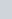7). Mile – hour is multiplied to make foot – second =8). meter – second is multiplied to make km – hour =9). km – hour is multiplied to make meter – second =10). If a man covers the distance between two fixed places with a speed of a km/h, he reaches t1 hour late, and when he travels at a speed of b km/h, he reaches t2 hours early, then the distance between the two places =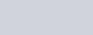11). If a man walks at a km/h, he reaches his destination t1 hour late, next time he increases his speed by b km/h, he reaches t2 hour late, then the distance covered by him =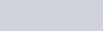12). Two persons X and Y start to cover a distance D km from point A to B with the speed of x km/h and y km/h respectively, if Y reaches point B and returns soon, and meets X at point C, then-
* distance traveled by X =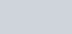* distance traveled by Y =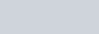13). A policeman sees a thief at a distance of d km, when he starts chasing the thief, the thief also starts running, if the speed of the thief and the policeman is a km/h and b km/h respectively, (b> a), then the distance traveled by the thief before being caught=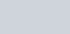14). If the ratio of the speeds of two objects is x:y, then the ratio of time taken by them is y:x

15). If an object covers two equal distances with speeds of x km/h and y km/h respectively, then the average speed for the whole journey is =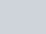16). If a person walks at the speed x/y of his original speed, and reaches the destination t time before/after, then the time taken to reach the destination with the new speed =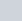If (x>y), and, if (y>x)

17). If two objects move in the same direction with a speed of a km/h and b km/h (a>b), then their relative speed = (a-b) km/h

18). If two objects move in opposite directions with a speed of a km/h and b km/h, (a>b), then their relative speed = (a+b) km/h

19). If an object P departs from A to B and another object Q leaves from B to A at the same time and takes t1 and t2 respectively to reach B and A respectively from the time after meeting, Then P’s speed / Q’s speed =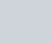20). When a train crosses a stationary object, tree, pole, or person, then = The train has to travel a distance equal to its own length.

21). When a train crosses a long object such as a platform, bridge, or any other train, then =  the train has to cover a distance equal to the sum of the length of the object and itself.

22). A train crosses a person in t1 time and a platform of length x in time t2, then the length of the train =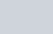23). Time is taken by the train to cross a pole or tree =24). time is taken by the vehicle to cross a bridge or platform =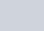Where l is the length of the train/train, and L is the length of the object/platform.
25). If the car is going in the same direction then –

*.Time is taken to catch the slow-moving train =
(Distance covered by it in extra time)/(difference of their speed)

*. time taken to cross each other =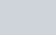26). If a train covers a distance D1 with a speed of x1 km/h, a distance D2 with a speed of x2 km/h and a distance D3 at a speed of x3 km/h, then their average speed is =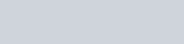27). If the speed is taken to be constant, then the distance covered by a person is proportional to the time, then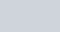28). If time is taken as a constant, then the distance covered by a person is proportional to the speed, then29). If distance is kept constant, then speed is inversely proportional to time, then